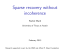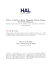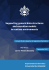# High–performance graph algorithms from parallel sparse matrices

## Transcription

High–performance graph algorithms from parallel sparse matrices
```High–performance graph algorithms from
parallel sparse matrices
John R. Gilbert1∗ , Steve Reinhardt2 , and Viral B. Shah1
1
University of California, Santa Barbara
{gilbert,viral}@cs.ucsb.edu†
2
Silicon Graphics Inc.
[email protected]
Abstract. Large–scale computation on graphs and other discrete structures is becoming increasingly important in many applications, including computational biology, web search, and knowledge discovery. High–
performance combinatorial computing is an infant field, in sharp contrast
with numerical scientific computing.
We argue that many of the tools of high-performance numerical computing – in particular, parallel algorithms and data structures for computation with sparse matrices – can form the nucleus of a robust infrastructure for parallel computing on graphs. We demonstrate this with an
implementation of a graph analysis benchmark using the sparse matrix
infrastructure in Star-P, our parallel dialect of the Matlab programming language.
1
Introduction
High performance applications increasingly combine numerical and combinatorial algorithms. Past research on high performance computation has focused
mainly on numerical algorithms, and we have a rich variety of tools for high performance numerical computing. On the other hand, few tools exist for large–scale
combinatorial computing.
Our goal is to build a general set of tools to allow scientists and engineers
develop applications using modern numerical and combinatorial tools with as
little effort as possible. Sparse matrix computations allow structured representation of irregular data structures, decompositions, and irregular access patterns
in parallel applications.
Sparse matrices are a convenient way to represent graphs. Since sparse matrices are first class citizens in Matlab and many of its parallel dialects, it is
natural to use the duality between sparse matrices and graphs to develop a rich
infrastructure for numerical and combinatorial computing.
∗
†
This author’s work was partially supported by Silicon Graphics Inc.
These authors’ work was partially supported by the Air Force Research Laboratories
under agreement number AFRL F30602-02-1-0181 and by the Department of Energy
under contract number DE-FG02-04ER25632.
Sparse matrix operation
Graph operation
G = sparse (U, V, W)
Construct a graph from an edge list
[U, V, W] = find (G)
Obtain the edge list from a graph
vtxdeg = sum (spones(G))
Vertex degrees for an undirected graph
indeg = sum (spones(G))
Indegrees for a directed graph
outdeg = sum (spones(G), 2) Outdegrees for a directed graph
N = G(i, :)
Find all neighbors of vertex i
Gsub = G(subset, subset)
Extract a subgraph of G
G(i, j) = W
Add or modify a graph edge
G(i, j) = 0
Delete a graph edges
G(I, I) = []
Remove vertices from a graph
G = G(perm, perm)
Permute vertices of a graph
reach = G * start
Table 1. Correspondence between some sparse matrix and graph operations.
2
Sparse matrices and graphs
Every sparse matrix problem is a graph problem and every graph problem is
a sparse matrix problem. We discuss some of the basic design principles to be
aware of when designing a comprehensive infrastructure for sparse matrix data
structures and algorithms in our earlier work [5, 10]. The same principles apply
to efficient operations on large sparse graphs.
1. Storage for a sparse matrix should be θ(max(n, nnz))
2. An operation on sparse matrices should take time approximately proportional to the size of the data accessed and the number of nonzero arithmetic
operations on it.
A graph consists of a set of vertices V , connected by edges E. A graph can
be specified by tuples (u, v, w) – this means that there exists a directed edge
of weight w from vertex u to vertex v. This is the same as a nonzero w at
location (u, v) in a sparse matrix. According to Principle 1, the storage required
is θ(|V |+|E|). An undirected graph is represented by a corresponding symmetric
sparse matrix.
A correspondence between sparse matrix operations and graph operations is
listed in Table 1. The basic design principles silently come into play in all cases.
Consider breadth first search (BFS). A BFS can be performed by multiplying
a sparse matrix G with a sparse vector x. The simplest case is doing a BFS
starting from vertex i. In this case, we set x(i) = 1, all other elements being
zeros. y = G ∗ x simply picks out column i of G which contains the neighbors of
vertex i. If we repeat this step again, the multiplication will result in a vector
which is a linear combination of all columns of G corresponding to the nonzero
elements in vector x, or all vertices that are up to 2 hops away from vertex i. We
can also do several independent BFS searches simultaneously by using sparse
matrix sparse matrix multiplication . Instead of starting with a vector, we
start with a matrix, with one nonzero in each column at some row j, where j is
the starting vertex. So, we have Y = G ∗ X, where each column of J contains
the results of performing an independent BFS. Sparse matrix multiplication can
be thought of as simply combining columns of G, and Principle 2 assures us
that each of these indexing operations take time proportional to the number of
nonzeros in that column. As a result, the time complexity of performing BFS
using operations on sparse matrices is the same as that obtained by performing
operations on other efficient graph data structures.
3
An example: SSCA #2 graph analysis benchmark
The SSCAs (Scalable Synthetic Compact Applications) are a set of benchmarks
designed to complement existing benchmarks such as the HPL  and the NAS
parallel benchmarks . Specifically, SSCA #2  is a compact application that
has multiple kernels accessing a single data structure (a directed multigraph with
weighted edges). The data generator generates an edge list in random order for a
multigraph of sparsely connected cliques as shown in Figure 1. The four kernels
are as follows:
1.
2.
3.
4.
Kernel 1: Create a data structure for further kernels.
Kernel 2: Search graph for a maximum weight edge.
Kernel 3: Perform breadth first searches from a set of start vertices.
Kernel 4: Recover the underlying clique structure from the undirected graph.
The benchmark spec is still not finalized. We describe our implementation of
version 1.1 (integer only) of the spec in this paper.
Fig. 1. SSCA #2 graph (a) Conceptual (b) Plotted with Fiedler co–ordinates.
3.1
Scalable data generator
The data generator is the most complex part of our implementation. It generates
edge tuples for subsequent kernels. No graph operations are performed at this
stage. The input to the data generator is a scale parameter, which indicates the
size of the graph being generated. The resulting graph has 2scale vertices, with
a maximum clique size of b2scale/3 c, a maximum of 3 edges with the same endpoints, and a probability of 0.2 that an edge is directional. The vertex numbers
are randomized, and a randomized ordering of the edge tuples is presented to
the subsequent kernels. Our implementation of the data generator closely follows
the pseudocode published in the spec.
3.2
Kernel 1
Kernel 1 creates a read–only data structure that is used by subsequent kernels.
We create a sparse matrix corresponding to each layer of the multigraph. The
multigraph has 3 layers, since there is a maximum of 3 parallel edges between any
two vertices in the graph. Matlab provides several ways of constructing sparse
matrices, sparse() being one of them. It takes as its input a list of 3–tuples (i, j, wij ). Its output is a sparse matrix with a nonzero wij in every location (i, j)
specified in the input. Figure 2 shows a spy plot of one layer of the input graph.
0
100
200
300
400
500
600
700
800
900
1000
0
200
400
600
nz = 7464
800
1000
Fig. 2. Matlab spy plot of the input graph
3.3
Kernel 2
In kernel 2, we search the graph for edges with maximum weight. find() is the
inverse of sparse(). It returns all nonzeros from a sparse matrix as a list of
3–tuples. We then use max() to find the maximum weight edge.
3.4
Kernel 3
In kernel 3, we perform breadth first searches from a given set of starting points.
We use sparse matrix–matrix multiplication to perform all breadth first searches
simultaneously from the given starting points. Let G be the adjacency matrix
representing the graph and S be a matrix corresponding to the starting points. S
has one non–zero in each column for every starting point. Breadth first search is
performed by repeatedly multiplying G with S: Y = G ∗ X. We perform several
breadth first searches simultaneously by using sparse matrix–matrix multiplication. Star-P stores sparse matrices by rows, and parallelism is achieved by each
processor computing some rows in the product [10, 9].
3.5
Kernel 4
Kernel 4 is the most interesting part of the benchmark. It can be considered
to be a partitioning problem or a clustering problem. We have several implementations of kernel 4 based on spectral partitioning (Figure 1), “seed growing”
(Figure 3), and “peer pressure” algorithms. The peer pressure and seed growing
implementations scale better than the spectral methods, as expected. We now
demonstrate how we use the infrastructure described above to implement kernel
4 in a few lines of Matlab. Figure 3 shows a spy plot of the undirected graph
after clustering. The clusters show up as dense blocks along the diagonal.
0
0
100
50
200
300
100
400
500
150
600
200
700
800
250
900
1000
300
0
200
400
600
nz = 8488
800
1000
0
50
100
150
nz = 1934
200
250
300
Fig. 3. (a) Clusters in full graph (b) Magnification around the diagonal
Our seed growing algorithm (Figure 4) starts with picking a small set of seeds
(about 2% of the total number of vertices) randomly. The seeds are then grown
so that each seed claims all vertices reachable by at least k paths of length 1
or 2. This may cause some ambiguity, since some vertices might be claimed by
multiple seeds. We tried picking an independent set of vertices from the graph by
performing one round of Luby’s algorithm  to keep the number of such ambiguities as low as possible. However, the quality of clustering remained unchanged
when we use random sampling. We used a simple approach for disambiguation
– the lowest numbered cluster claiming a vertex got it. We also experimented
with attaching singleton vertices to nearby clusters to improve the quality of
clustering.
% J is a sparse matrix with one seed per column.
J = sparse (seeds, 1:nseeds, 1, n, nseeds);
J = G * J;
J = J + G*J;
J = J > k;
% Vertices reachable with 1 hop.
% Vertices reachable with 1 or 2 hops.
% Vertices reachable with at least k paths of 1 or 2 hops.
Fig. 4. Breadth first parallel clustering by seed growing.
Our peer pressure algorithm (Figure 5) starts with a subset of vertices designated as leaders. There has to be at least one leader neighboring every vertex
in the graph. This is followed with a round of voting where every vertex in the
graph elects a leader, selecting a cluster to join. This does not yet yield good
clustering. Each vertex now looks at its neighbors and switches its vote to the
most popular leader in its neighborhood. This last step is crucial, and in this
case, it recovers more than 95% of the original clique structure of the graph.
We experimented with different approaches to select leaders. At first, it
seemed that a maximal independent set of vertices from the graph was a natural
way to pick leaders. In practice, it turned out that simple heuristics (such as
the highest numbered neighbor) gave equally good clustering. We also experimented with different numbers of voting rounds. The marginal improvement in
the quality of clustering was not worth the additional computation required.
% IS is the independent set. Find all neighbors in the IS.
neighbors = G * sparse(IS, IS, 1, n, n);
% Each vertex chooses a random neighbor in the independent set.
R = sprand (neighbors);
[ignore vote] = max (R, [], 2);
% Collect neighbor votes and join the most popular cluster.
[I, J] = find (G);
S = sparse (I, vote(J), 1, n, n);
[ignore cluster] = max (S, [], 2);
Fig. 5. Parallel clustering by peer pressure
We used Star-P  for our implementation. Star-P is a parallel implementation of the Matlab language with global array semantics. We expect it to
be straightforward to port to any other global–array parallel dialect of Matlab, such as pMatlab  or Mathworks Parallel Matlab . We present a
basic performance analysis of our implementation in Section 5. We will include
a detailed performance analysis of our implementation in a forthcoming journal
version of our paper.
4
Visualization of large graphs
Graphics and visualization are a key part of an interactive system such as Matlab. The question of how to effectively visualize large datasets in general, especially large graphs, is still unsolved. We successfully applied methods from
numerical computing to come up with meaningful visualizations of the SSCA
#2 graph.
One way to compute geometric co–ordinates for the vertices of a connected
graph is to use Fiedler co–ordinates for the graph. Figure 1 shows the Fiedler embedding of the SSCA #2 graph. In the 2D case, we use the eigenvectors (Fiedler
vectors) corresponding to the first two non–zero eigenvalues as co–ordinates for
the graph vertices in a plane.
120
10
90
20
30
60
40
50
30
60
0
10
20
30
40
50
60
Matrix nr = 1024, nc = 1024, nnz = 7144
Bucket nnz: max = 120, min = 0, avg = 1.74414, total = 7144, max/avg = 69
Fig. 6. SSCA #2 graph: (a) 3D visualization (b) density plot (spyy)
For 3D visualization of the SSCA #2 graph, we start with 3D Fiedler coordinates. We model the graph as particles on the surface of a sphere. There
is a repulsive force between all particles, inversely proportional to the distance
between them. If r is the distance between two particles, the forcing function we
use is 1/r. Since these particles repel each other on the surface of a sphere, we
expect them to spread around and occupy the entire surface of the sphere. Since
there are cliques in the original graph, we expect clusters of particles to form on
the surface of the sphere. At each timestep, we compute a force vector between
all pairs of particles. Each particle is then displaced some distance based on its
force vector. All displaced particles are projected back onto the sphere at the
end of each timestep.
This algorithm was used to generate Figure 6. In this case, we simulated
256 particles and the system was evolved for 20 timesteps. It is important to
first calculate the Fiedler co–ordinates. Our effort to use random co–ordinates
resulted in a meaningless picture. We used PyMOL  to render the graph.
We also developed a version of spy() suitable for visualization of large graphs
- spyy(). Large sparse graphs are often stored remotely (for example, on a
Star-P server). It is impracatical to transfer the entire graph to the frontend
for display. We create a density plot of the sparse matrix, and only transfer
the image to the frontend. We implemented spyy() completely in the Matlab
language. It uses parallel sparse matrix multiplication to build the density plot on
the backend. A spyy plot can also be thought of as a two dimensional histogram.
Figure 6 shows a spyy plot of the SSCA #2 graph after clustering.
5
Experimental Results
We ran our implementation of SSCA #2 (ver 1.1, integer only) in Star-P. The
Matlab client was run on a generic PC. The Star-P server was run on an
SGI Altix with 128 Itanium II processors with 128G RAM (total, non-uniform
memory access). We used a graph generated with scale 21. This graph has
2, 097, 152 vertices. The multigraph has 320, 935, 185 directed edges, whereas
the undirected graph corresponding to the multigraph has 89, 145, 367 edges.
There are 32, 342 cliques in the graph, the largest of them having 128 vertices.
There are 88, 933, 116 undirected edges within cliques, and 212, 251 undirected
edges between cliques in the input graph for kernel 4. The results are presented
in Fig. 7.
Fig. 7. SSCA #2 v1.1 execution times (Star-P, Scale=21)
Operation
Source LOC Total line counts
Data generator
176
348
Kernel 1
25
63
Kernel 2
11
34
Kernel 3
23
48
Kernel 4 (spectral)
22
70
Kernel 4 (seed growing)
55
108
Kernel 4 (peer pressure)
6
29
Table 2. Line counts for Star-P implementation of SSCA#2. The “Source LOC”
column counts only executable lines of code, whereas the “Line counts” column counts
the total number of lines including comments and whitespace.
Although it is not required by the spec, our data generator also scales very
well. A lot of time is spent in kernel 1, where data structures for the subsequent
kernels are created. The majority of the time is spent in searching the input
triples for duplicates, since the input graph is a multigraph. Kernel 1 creates
several sparse matrices using sparse(), each corresponding to a layer in the
multigraph. Time spent in kernel 1 also scales very well with the number of
processors. Time spent in Kernel 2 also scales as expected.
Kernel 3 does not show speedups at all. Although, all the breadth first
searches are performed in parallel, the process of subgraph extraction for each
starting point creates a lot of traffic between the Star-P client and the Star-P
server - which were physically in different states. This client server communication time ends up dominating over the computation time. We will minimize this
overhead by vectorizing all of kernel 3 in a future release.
Kernel 4, the non–trivial part of the benchmark, actually scales very well.
We present results for our best performing implementation of kernel 4, which
uses the seed growing algorithm.
The evaluation criteria for the SSCAs also include software engineering metrics such as code size, readability, maintainability etc. Our implementation is
extremely concise. We provide the source lines of code (SLOC) for our implementation in Table 2. We also provide absolute line counts which include blank
lines and comments, as we believe these to be crucial for code readability and
maintainability. Our implementation runs without modification in sequential
Matlab, making it easy to develop and debug on the desktop before deploying
on a parallel platform.
6
Concluding remarks
We have run the full SSCA #2 benchmark (spec v0.9, integer only) on graphs
with 227 = 134 million vertices on the SGI Altix. We have also manipulated
extremely large graphs (1 billion vertices and 8 billion edges) on an SGI Altix
with 256 processors using Star-P.
We demonstrate a robust, scalable way to manipulate large graphs by representing them with sparse matrices. Although it may be possible to achieve higher
performance with different data structures and distributions, it is extremely hard
to design a general purpose system which can support such a variety of representations, and the resulting combinatorial explosion of interactions between
them. This is why Star-P has only one representation for sparse matrices .
This allows for a robust, scalable, well–tuned implementation of sparse matrix
algorithms, and hence, operations on graphs.
Note that the codes in Figure 4 and Figure 5 are not pseudocodes, but
actual code excerpts from our implementation. Although the code fragments
look very simple and structured, the computation is anything but. All operations
are on sparse matrices, resulting in highly irregular communication patterns on
irregular data structures. We conclude that sparse matrix operations provide a
convenient language to efficiently manipulate large graphs.
References
1. D. A. Bader, K. Madduri, J. R. Gilbert, V. Shah, J. Kepner, T. Meuse, and A. Krishnamurthy. Designing scalable synthetic compact applications for benchmarking high productivity computing systems. Cyberinfrastructure Technology Watch,
2(4B), November 2006.
2. D. H. Bailey, E. Barszcz, J. T. Barton, D. S. Browning, R. L. Carter, D. Dagum,
R. A. Fatoohi, P. O. Frederickson, T. A. Lasinski, R. S. Schreiber, H. D. Simon,
V. Venkatakrishnan, and S. K. Weeratunga. The NAS parallel benchmarks. The
International Journal of Supercomputer Applications, 5(3):63–73, Fall 1991.
3. Warren L. DeLano. The PyMOL molecular graphics system. 2006. DeLano Scientific LLC, San Carlos, CA, USA. http://www.pymol.org/.
4. J. J. Dongarra. Performance of various computers using standard linear equations
software in a Fortran environment. In Walter J. Karplus, editor, Multiprocessors
and array processors: proceedings of the Third Conference on Multiprocessors and
Array Processors: 14–16 January 1987, San Diego, California, pages 15–32, San
Diego, CA, USA, 1987. Society for Computer Simulation.
5. J. R. Gilbert, C. Moler, and R. Schreiber. Sparse matrices in MATLAB: Design
and implementation. SIAM J. on Matrix Anal. Appl., 13(1):333–356, 1992.
6. P. Husbands and C. Isbell. MATLAB*P: A tool for interactive supercomputing.
SIAM Conference on Parallel Processing for Scientific Computing, 1999.
7. Michael Luby. A simple parallel algorithm for the maximal independent set problem. SIAM J. Comput., 15(4):1036–1053, 1986.
8. C. B. Moler. Parallel matlab. Householder Symposium on Numerical Algebra, 2005.
9. Christopher Robertson. Sparse parallel matrix multiplication. M.S. Project, Department of Computer Science, UCSB, 2005.
10. Viral Shah and John R. Gilbert. Sparse matrices in Matlab*P: Design and implementation. In Luc Bougé and Viktor K. Prasanna, editors, HiPC, volume 3296 of
Lecture Notes in Computer Science, pages 144–155. Springer, 2004.
11. Nadya Travinin and Jeremy Kepner. pMatlab parallel matlab library. Submitted
to International Journal of High Performance Computing Applications, 2006.
```

### High–performance graph algorithms from parallel sparse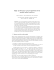structures and decompositions, and irregular access patterns in parallel applications. Every sparse matrix problem is a graph problem and every graph problem is a sparse matrix problem. We reiterat...

### Magret du canard, foie gras, cassoulet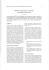### Fiter: Greedy Bloggers for a Picky Audience Avner May, Augustin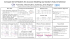### TexPoint fonts used in EMF.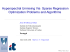### TÉCNICAS Y DISTRIBUCIONES TIEMPO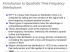### Intrusion Detection Systems What To Observe? What To Observe?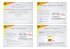### 1. Basic Concepts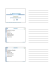### PDF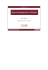### Sparse direct linear solvers Woudschoten conference on Parallel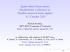### Sparse recovery without incoherence